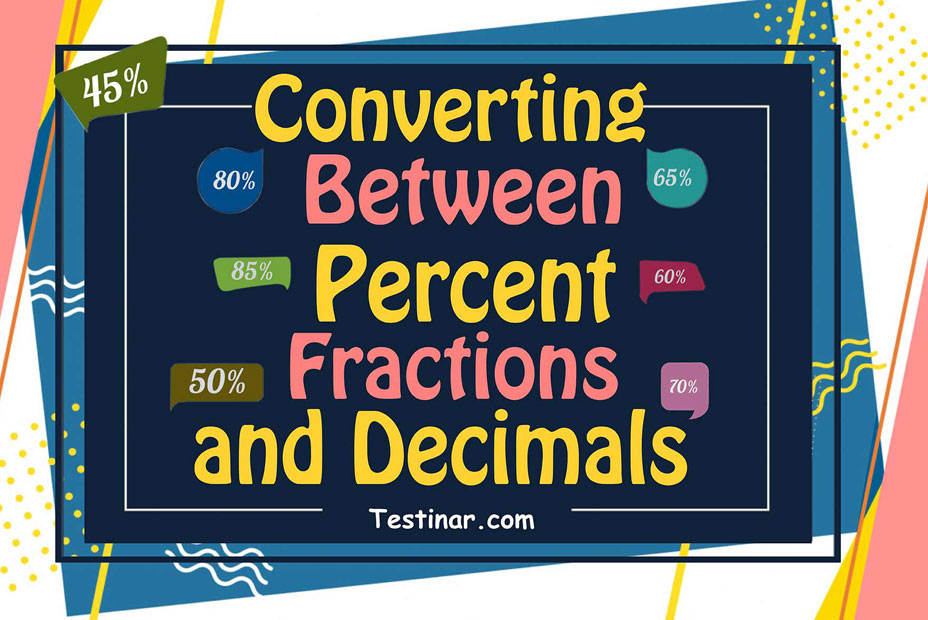## How to Convert Between Percent, Fractions, and Decimals?

Did you know that the term percent was derived from the Latin word “per centum?” Also, if you break down the word “percent” into two words “per” and “cent” the meaning becomes quite evident, it means by hundred. So, we can say that percentages are nothing but fractions whose denominators would always be $$100$$.
So, we can say that in case of a percentage, $$100$$ is considered as the whole quantity. For example, if there are $$45\%$$ computer books in a library, then that means that out of every $$100$$ books in that library, $$45$$ are of computer science. It can also be used as a ratio like $$45:100$$. Also, in fraction form, it would be denoted as $$\frac{45}{100}$$ .

### Calculation of Percentage

Now that we know what is percentage, let’s find out how to calculate it with ease. In percentage problems, we basically have to find the part/share of a whole in terms of $$100$$. This we can do in the following 2 ways.

• Firstly, we can apply the unitary method.
• Secondly, we take the fraction in consideration and change its denominator to $$100$$.

A thing to note is that the second method can only be effectively used when the denominator of the fraction is a factor of $$100$$. If this isn’t the case, we will always resort to the first method.
Now, there is a very interesting formula to calculate the part, whole, and percentage.
This goes as $$part \ = \ \frac{percent}{100} \times whole$$
So, suppose in some numerical, you are asked to find out the separate entities like part or percent or even whole, then you can resort to this formula to find the individual answers.

### Converting a Fraction/Decimal to a Percent

A fraction is converted to a percent by first converting it to a decimal by dividing the numerator by the denominator of the fraction in question. Following that, once you've obtained the decimal, simply multiply it by $$100$$ to obtain the percentage.
Ex: $$\frac{1}{4} \ = \ 0.25 \ = \ 0.25 \times 100 \ = \ 25\%$$
To convert a percentage into fraction, just divide the percentage by $$100$$ and then simplify (if possible) to get the fraction.
Ex: $$25$$ $$\%$$ $$= \ \frac{25}{100} \ = \ \frac{1}{4} \ = \ 0.25$$

### Exercises for Converting Between Percent, Fractions, and Decimals

1) $$0.355 \ =$$

2) $$0.52 \ =$$

3) $$\frac{40}{100} \ =$$

4) $$\frac{45}{100} \ =$$

5) $$\frac{80}{100} \ =$$

6) $$0.295 \ =$$

7) $$0.66 \ =$$

8) $$0.2 \ =$$

9) $$0.425 \ =$$

10) $$\frac{85}{100} \ =$$

1) $$0.355 \ = \ \color{red}{35.5\% }$$
2) $$0.52 \ = \ \color{red}{52\% }$$
3) $$\frac{40}{100} \ = \color{red}{40 \ \div \ 100 \ = \ 0.4}$$
4) $$\frac{45}{100} \ = \color{red}{45 \ \div \ 100 \ = \ 0.45}$$
5) $$\frac{80}{100} \ = \color{red}{80 \ \div \ 100 \ = \ 0.8}$$
6) $$0.295 \ = \ \color{red}{29.5\% }$$
7) $$0.66 \ = \ \color{red}{66\% }$$
8) $$0.2 \ = \ \color{red}{20\% }$$
9) $$0.425 \ = \ \color{red}{42.5\% }$$
10) $$\frac{85}{100} \ = \color{red}{85 \ \div \ 100 \ = \ 0.85}$$

## Converting Between Percent, Fractions, and Decimals Quiz

### The Most Comprehensive ASVAB Math Preparation Bundle

$76.99$36.99

### GED Math for Beginners 2022

$28.99$14.99

### PSAT Math for Beginners

$24.99$14.99

### ASVAB Math Full Study Guide

$25.99$13.99